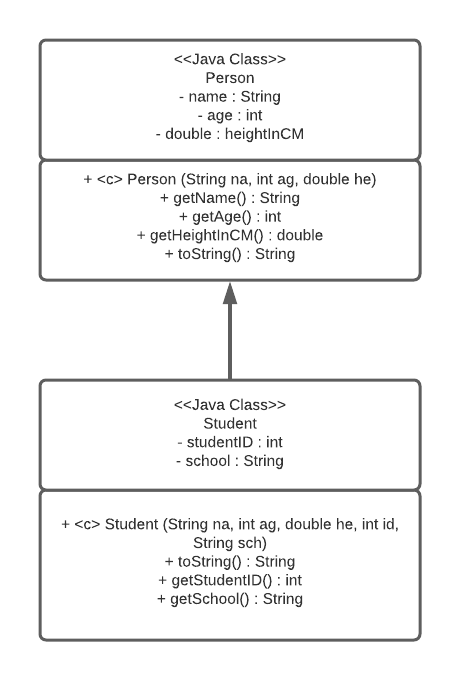# X433: Create ToString

For this question, assume the following implementation of the class Person and the following UML diagram

#### Person Class Implementation

``````public class Person{
private String name;
private int age;
private double heightInCM;

public Person (String na, int ag, double he) {
name  = na;
age = ag;
heightInCM = he;
}

public String getName() {
return name;
}
public int getAge() {
return age;
}
public double getHeightInCM() {
return heightInCM;
}

@Override
public String toString() {
return getName()
+ ":\n Age: " + getAge()
+ "\n Height: " + getHeightInCM() + " cm";
}
}
``````

#### UML diagramImplement a toString method in the Student class that will override the one in the Person class. The method Student.toString() should include all the information from Person.toString() plus studentID and school.

For example, given the following Person object: `Person p1 = new Person("Dan", 25, 125)`

`p1.toString()` will return the following String:

``````Dan_25_12
``````

Given the following Student object: `Student s1 = new Student("Dan", 25, 125, 222, "Virginia Tech")`

`s1.toString()` should return the following String:

``````Dan_25_125_222_Virginia Tech
``````